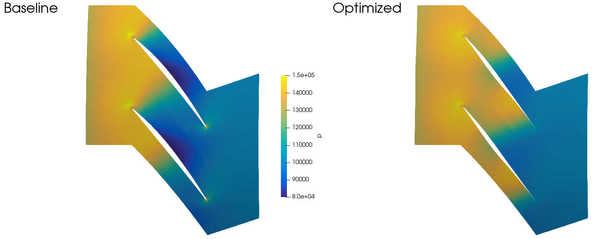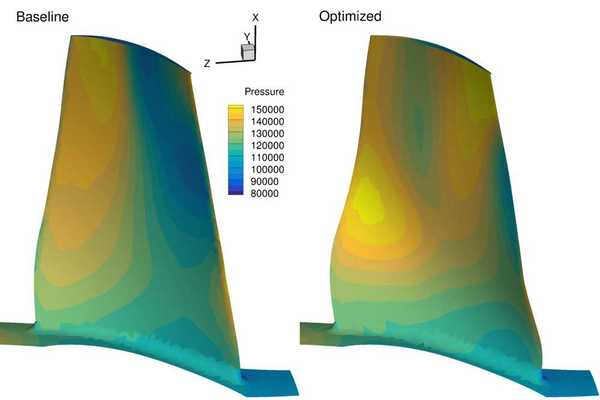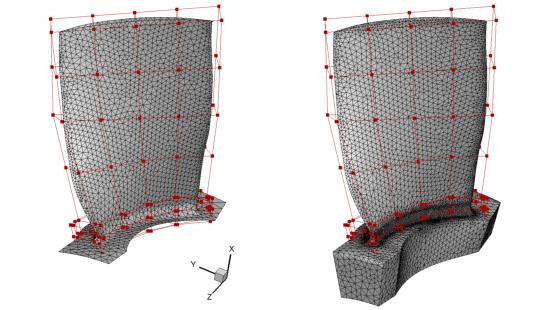# Axial compressor rotor¶

NOTE: Before running this case, please read the instructions in NACA0012 airfoil incompressible to get an overall idea of the DAFoam optimization setup.

This is an aerodynamic shape optimization case for the Rotor67 axial compressor. The summary of the case is as follows:

Case: Axial compressor aerodynamic optimization at transonic conditions
Geometry: Rotor67
Objective function: Torque
Design variables: 80 FFD points moving in the y and z directions
Constraints: Constant mass flow rate and total pressure ratio
Tip Mach number: 1.38
Rotation speed: -1680 rad/s
Mesh cells: 60K

The configuration files are available at Github. To run this case, first source the DAFoam environment (see Tutorials). Then you can go into the run folder and run:

```./Allrun.sh 8
```

The optimization progress will then be written in the log.opt file. NOTE, we recommend running this case on an HPC system using at least 8 CPU cores.

For this case, the optimization was run for 20 steps, see the following figure. The baseline design has C_M=0.08574, m=1.733 m^3/s, p1/p0=1.463, and the optimized design has C_M=0.0782, m=1.625 m^3/s, p1/p0=1.460.The mesh and FFD points are as follows. We use ICEM to generate the triangular meshes and FFD points.